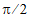# NMath User's Guide

33.1 Finding Function Roots Without Calculating the Derivative (.NET, C#, CSharp, VB, Visual Basic, F#)

NMath provides several classes that implement the interface, and find roots of univariate functions using only function evaluations:

Class finds roots of univariate functions using the secant method. The secant method assumes that the function is approximately linear in the local region of interest and uses the zero-crossing of the line connecting the limits of the interval as an estimate of the root. The function is evaluated at the estimate, a new line is formed, and the process is repeated.

Class finds roots of univariate functions using Ridders' Method. Ridders' Method first evaluates the function at the midpoint of the interval, then factors out the unique exponential function which turns the residual function into a straight line.

Class finds roots of univariate functions using the zeroin() root finder published originally in Computer Methods for Mathematical Computations by Forsythe, Malcolm and Moler in 1977. This class is similar to MATLAB's fzero() function.

Instances are constructed by specifying an error tolerance and a maximum number of iterations, or by accepting the defaults for these values. For example, this code constructs a SecantRootFinder using the default tolerance and a maximum of 50 iterations:

Code Example – C# root finding

```int maxIter = 50;
```
```var finder = new SecantRootFinder( maxIter );
```

Code Example – VB root finding

```Dim MaxIter As Integer = 50
```
```Dim Finder As New SecantRootFinder(MaxIter)
```

Instances provide Find() methods for minimizing a given function within a given interval. For instance, the cosine function has a root at:

Code Example – C# root finding

```var f = new OneVariableFunction(
```
```  new Func<double, double>( Math.Cos ) );

```

```var finder = new RiddersRootFinder();
```
```double lower = 0;
```
```double upper = Math.PI;
```
```double root = finder.Find( f, lower, upper );
```

Code Example – VB root finding

```Dim F As New OneVariableFunction(
```
```  New Func(Of Double, Double)(AddressOf Math.Cos))

```

```Dim Finder As New RiddersRootFinder()
```
```Dim Lower As Double = 0
```
```Dim Upper As Double = Math.PI
```
```Dim Root As Double = Finder.Find(F, Lower, Upper)
```

Top

Top